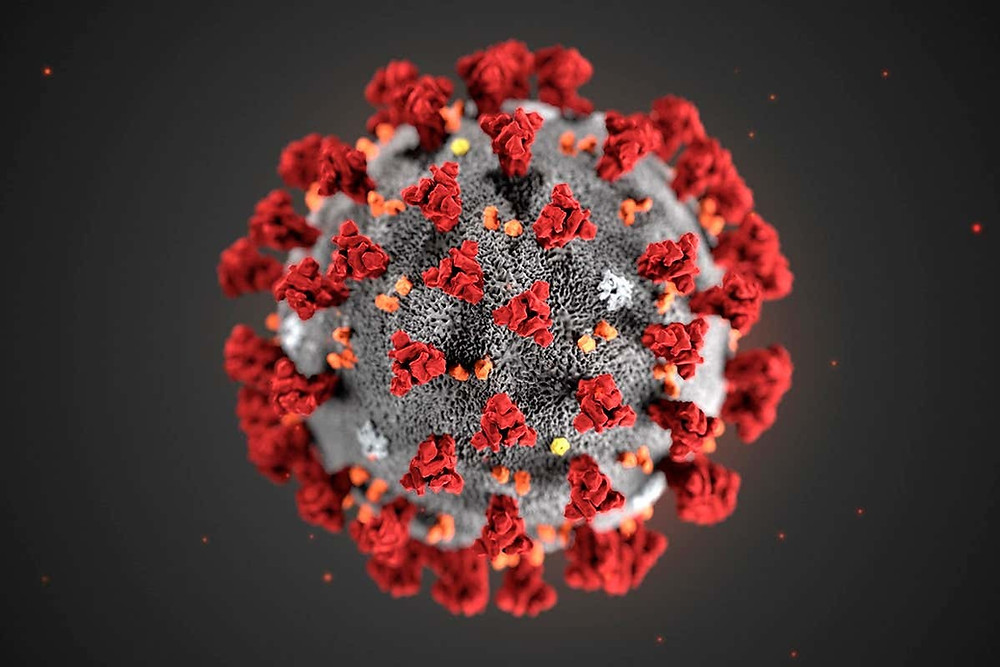Search

# Covid-19 Data Analysis in INDIA

Updated: Feb 1COVID-19, a novel coronavirus, is currently a major worldwide threat. It has infected more than five million people globally leading to thousands of deaths. In such grave circumstances, it is very important to predict the future infected cases to support the prevention of the disease and aid in the healthcare service preparation. Following that notion, we have developed a model and then employed it for time-series analysis of COVID-19 cases in India. The study indicates an ascending trend for the cases in the coming days.

In this blog, we'll see different types of analysis of the Corona Virus in India.

Dependencies

• Pandas

• Numpy

• Matplotlib

• Seaborn

• Plotly

• Folium

1. Importing The Libraries

```import pandas as pd
import matplotlib.pyplot as plt
%matplotlib inline
import seaborn as sns
import plotly.express as px
import plotly.graph_objects as go
import folium
from folium import plugins
plt.rcParams['figure.figsize'] = 10, 12
import warnings
warnings.filterwarnings('ignore')```

In this Blog, we need to import all the libraries with all the dependencies.

The next step is to import the CSV files.

`df= pd.read_csv('covid_19_india.csv')`

We've read the CSV file in the above lines of code.

`df.drop(['ConfirmedIndianNational','ConfirmedForeignNational'],axis=1,inplace=True)`

We've dropped the Unnecessary columns in the above lines of code.

## In this step, we will analyze some visualizations.In this above visualization, we can see 2 bar charts of total and cured legend. The X-axis represents the number of cases whereas y-axis represents the state. The total defines the number of total cases reported in that state holds red colour, whereas the cured defines the total number of people cured of coronavirus in that state holds green colour.

We can see that Maharastra holds the most number of total confirmed cases as well as the highest number of cured people whereas some Union-territories like Dadra Nagar Haveli holds the least number of cases.

## Let's Check/Visualize wrt Age.

`age_details=pd.read_csv('AgeGroupDetails.csv')`

Here we have imported the dataset AgegroupDetails in the data frame age_details.In the above figure, we can see the group-wise age distributions in a pie-chart. From the above figure we've concluded that the age group between 20-29 has reported the maximum number of cases, so we can say mostly the youths and after that, the age range between 30-39 has reported the second maximum number of cases.

## Gender-wise

`individual_details=pd.read_csv('IndividualDetails.csv')`we can see the gender difference in the number of cases, here we can see the % of males is more than females, the reason might be the number of males is more than females or the females are following the lockdown rules more precisely than females.

## ICMR Testing Details

`ICMR_labs=pd.read_csv('ICMRTestingLabs.csv')`

In these above lines of code, we have imported the dataset of ICMRtestinglabs.

```values = list(ICMR_labs['state'].value_counts())
names = list(ICMR_labs['state'].value_counts().index)

plt.figure(figsize=(15,10))
sns.set_color_codes("pastel")
plt.title('ICMR Testing Centers in each State', fontsize = 20)
sns.barplot(x= values, y= names,color = '#9370db');```IN this above fig we can see that Maharashtra has the most number of ICMR testing labs and it is pretty obvious also as that state reported the maximum number of cases.

## Let's check for all the states

``` all_state = list(df['State/UnionTerritory'].unique())
all_state.remove('Unassigned')
#all_state.remove('Nagaland#')
#all_state.remove('Nagaland')
latest = df[df['Date'] > '10-08-20']
state_cases = latest.groupby('State/UnionTerritory')['Confirmed','Deaths','Cured'].max().reset_index()
latest['Active'] = latest['Confirmed'] - (latest['Deaths']- latest['Cured'])
state_cases = state_cases.sort_values('Confirmed', ascending= False).fillna(0)
states =list(state_cases['State/UnionTerritory'][0:15])

states_confirmed = {}
states_deaths = {}
states_recovered = {}
states_active = {}
states_dates = {}

for state in states:
df = latest[latest['State/UnionTerritory'] == state].reset_index()
k = []
l = []
m = []
n = []
for i in range(1,len(df)):
k.append(df['Confirmed'][i]-df['Confirmed'][i-1])
l.append(df['Deaths'][i]-df['Deaths'][i-1])
m.append(df['Cured'][i]-df['Cured'][i-1])
n.append(df['Active'][i]-df['Active'][i-1])
states_confirmed[state] = k
states_deaths[state] = l
states_recovered[state] = m
states_active[state] = n
date = list(df['Date'])
states_dates[state] = date[1:]```
```colors_list = ['cyan','teal']
states = individual_details['detected_state'].unique()
if len(states)%2==0:
n_rows = int(len(states)/2)
else:
n_rows = int((len(states)+1)/3)
plt.figure(figsize=(14,60))

for idx,state in enumerate(states):
plt.subplot(n_rows,3,idx+1)
y_order = individual_details[individual_details['detected_state']==state]['detected_district'].value_counts().index
try:
g = sns.countplot(data=individual_details[individual_details['detected_state']==state],y='detected_district',orient='v',color=colors_list[idx%2],order=y_order)
plt.xlabel('Number of Cases')
plt.ylabel('')
plt.title(state)
plt.ylim(14,-1)
except:
pass
plt.tight_layout()
plt.show()

```The above figure shows the cases of all the states with their respective districts.

Analysis in a Nutshell

Coderarts
.mp4
MP4 • 24.81MB

Thank You!

Happy Coding

### Recent Posts

See All

#### Deploying Machine Learning Models in SageMaker - AWS Cloud.

Tel: (+91) 0120  4118730

Time :   10 : 00  AM -  08 : 00 PM IST